# Solution to the first MATLAB assignment

First we set some parameters that will be used over and over:
tStart=0;
tEnd=20;
y0=1/2;

## First, let's look with a small number of points

We compare the three different methods with a big stepsize.
N=40;
h=(tEnd-tStart)/N;
t=linspace(tStart,tEnd,N+1);
yEuler=zeros(1,N+1);
yEuler(1)=y0;
yEulerImproved=yEuler;
yRunge=yEuler;
yX=yExact(t);
for k=1:N
yEuler(k+1)=eulerStep(@f,t(k),yEuler(k),h);
yEulerImproved(k+1)=eulerImprovedStep(@f,t(k),yEulerImproved(k),h);
yRunge(k+1)=RKStep(@f,t(k),yRunge(k),h);
end
plot(t,yEuler,t,yEulerImproved,t,yRunge,t,yX,'k--')
xlabel('$t$')
ylabel('$y$')
legend('Euler','Improved','Runke-Kutta','Exact','Location',"eastoutside")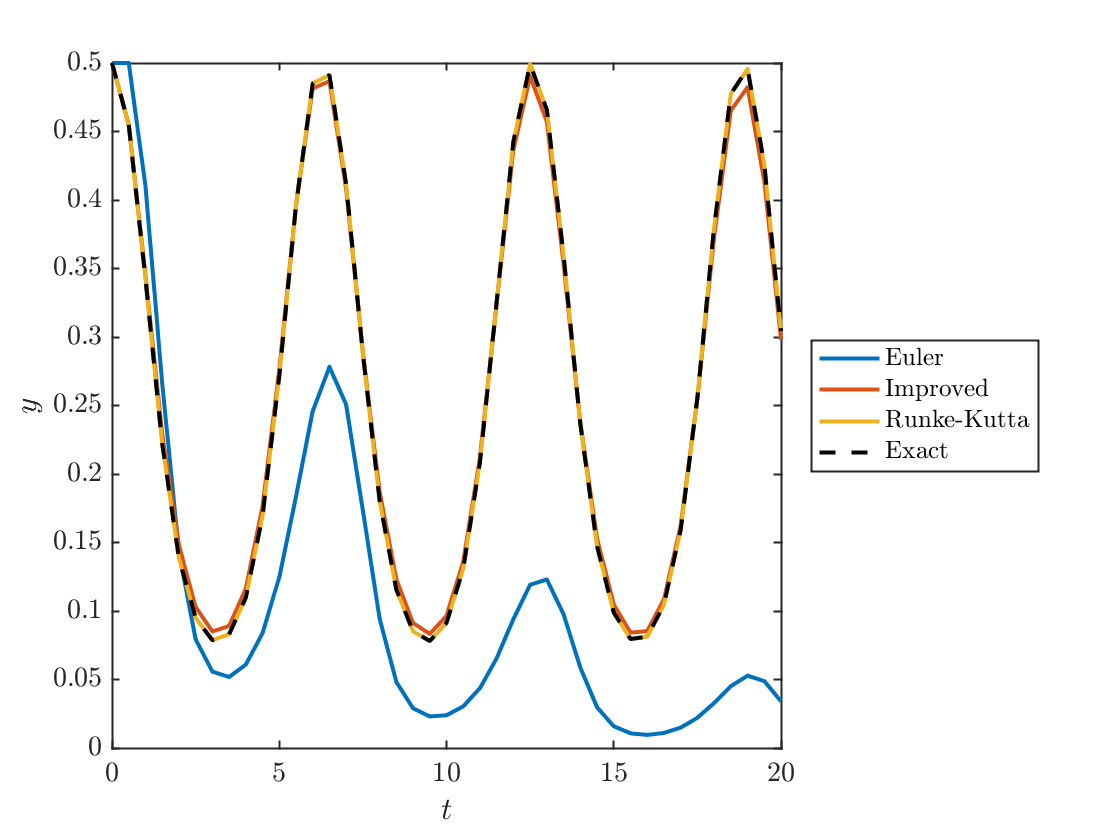## Plot the errors of the three methods

plot(t,yX-yEuler,t,yX-yEulerImproved,t,yX-yRunge)
xlabel('$t$')
ylabel('Error')
legend('Euler','Improved Euler','Runge-Kutta','location','northwest')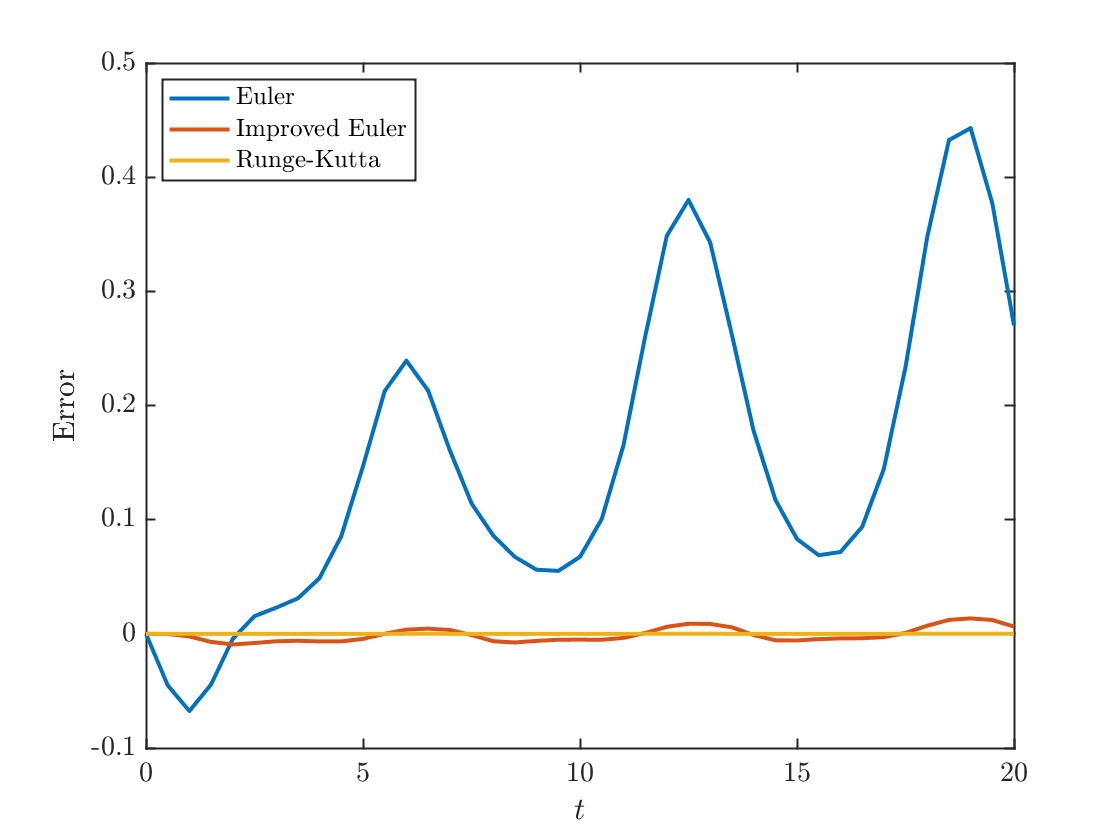errorEuler=abs(yX(end)-yEuler(end));
errorImproved=abs(yX(end)-yEulerImproved(end));
errorRunge=abs(yX(end)-yRunge(end));
fprintf('With 40 points, the final-time error is:\n')
With 40 points, the final-time error is:
fprintf('Euler method: %0.2d',errorEuler)
Euler method: 2.70e-01
fprintf('Improved Euler method: %0.2d',errorImproved)
Improved Euler method: 6.34e-03
fprintf('Runge-Kutta method: %0.2d',errorRunge)
Runge-Kutta method: 3.27e-05

## Experimentally find h small enough to make error below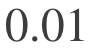We just keep cuttingh in half (doubling N) until we get below the threshold.
while errorEuler>0.01
N=2*N;
h=(tEnd-tStart)/N;
t=linspace(tStart,tEnd,N+1);
y=zeros(1,N+1);
y(1)=y0;
for k=1:N
y(k+1)=eulerStep(@f,t(k),y(k),h);
end
errorEuler=abs(yX(end)-y(end));
fprintf('N = %i, error=%0.3f\n',N,errorEuler)
end
N = 80, error=0.189 N = 160, error=0.110 N = 320, error=0.058 N = 640, error=0.030 N = 1280, error=0.015 N = 2560, error=0.007
plot(t,y,t,yExact(t),'--')
xlabel('$t$');ylabel('$y$')
legend('Euler','Exact')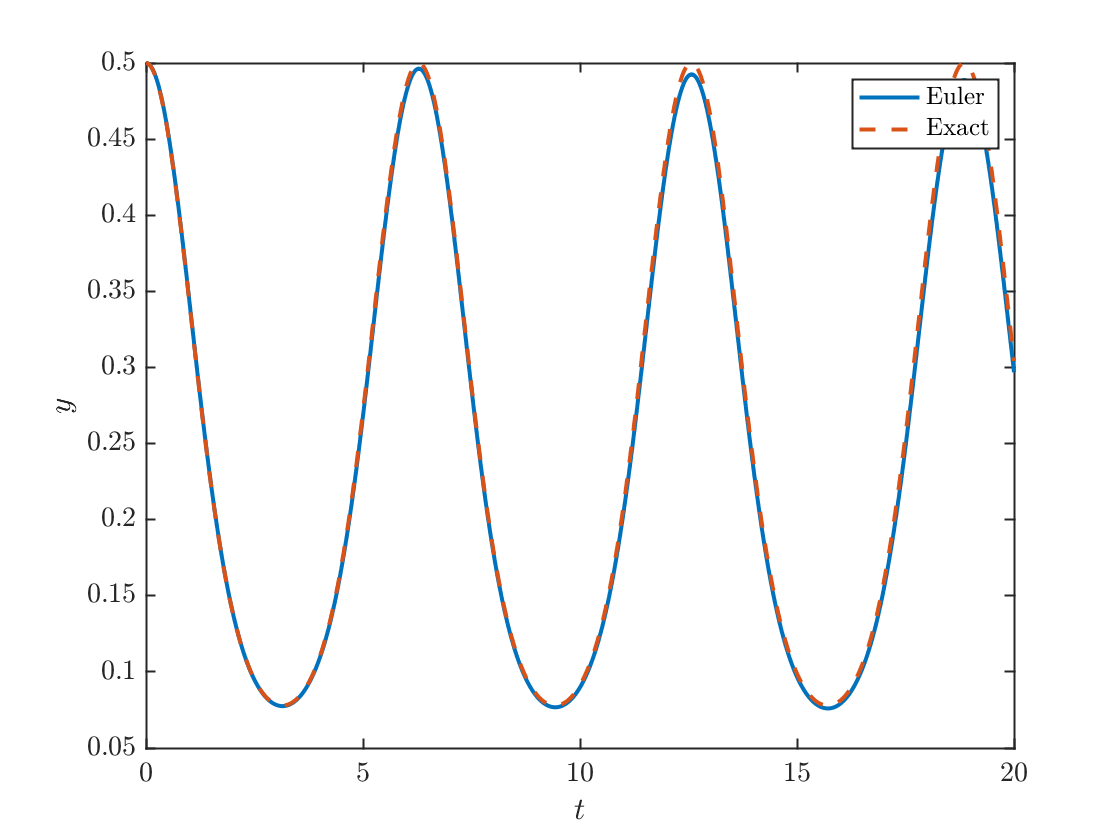That's good enough. Somewhere between 1280 and 2560 points, Euler's method returns an error below 0.01.

## For the improved Euler method, I found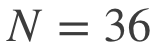points to be enough

Here I notice that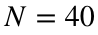was good enough in the initial experiment, so I just reduced it a bit at a time by hand until it failed.
N=36;
h=(tEnd-tStart)/N;
t=linspace(tStart,tEnd,N+1);
y=zeros(1,N+1);
y(1)=y0;
yX=yExact(t);
for k=1:N
y(k+1)=eulerImprovedStep(@f,t(k),y(k),h);
end
plot(t,y,t,yExact(t),'--')
xlabel('$t$');ylabel('$y$')
legend('Euler','Exact')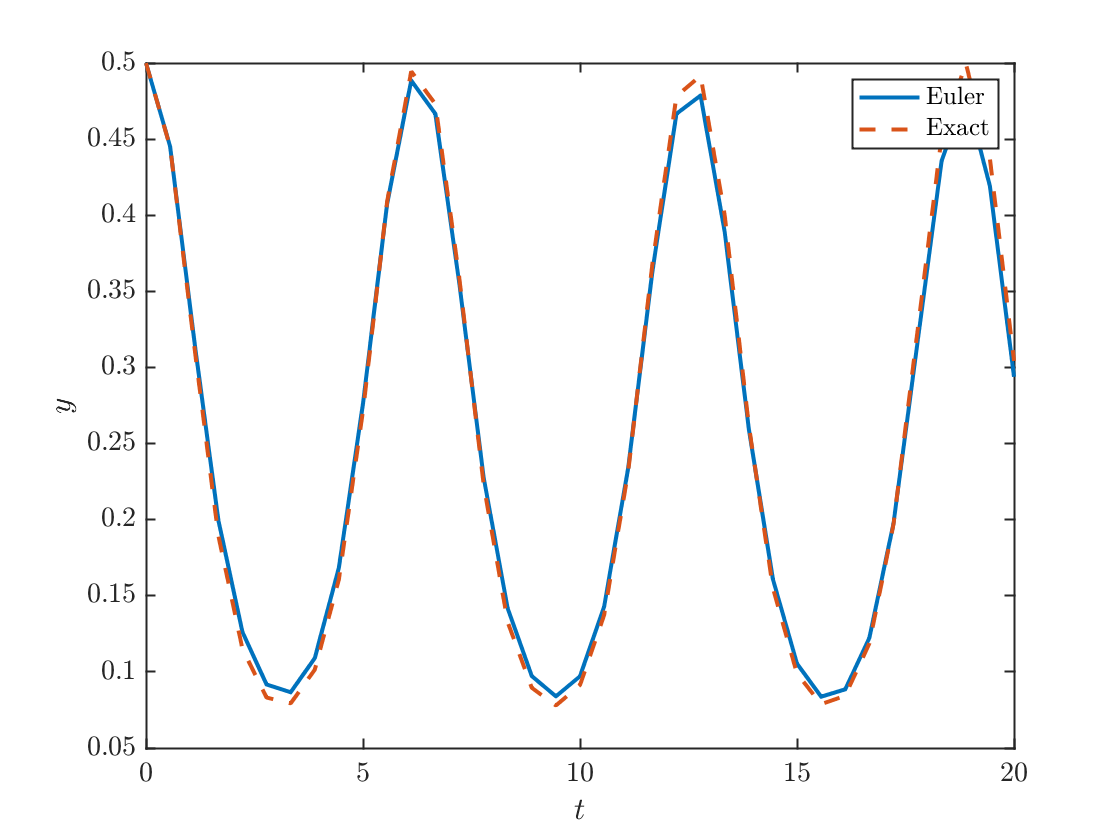error=abs(yX(end)-y(end));
fprintf('N = %i, error=%0.3f\n',N,error)
N = 36, error=0.010

## Runge Kutta can get away with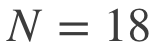points

Clearly this is not enough points to generate a nice smooth curve but the numbers it gives are very accurate.
N=18;
h=(tEnd-tStart)/N;
t=linspace(tStart,tEnd,N+1);
y=zeros(1,N+1);
y(1)=y0;
yX=yExact(t);
for k=1:N
y(k+1)=RKStep(@f,t(k),y(k),h);
end
plot(t,y,t,yExact(t),'--')
xlabel('$t$');ylabel('$y$')
legend('Euler','Exact')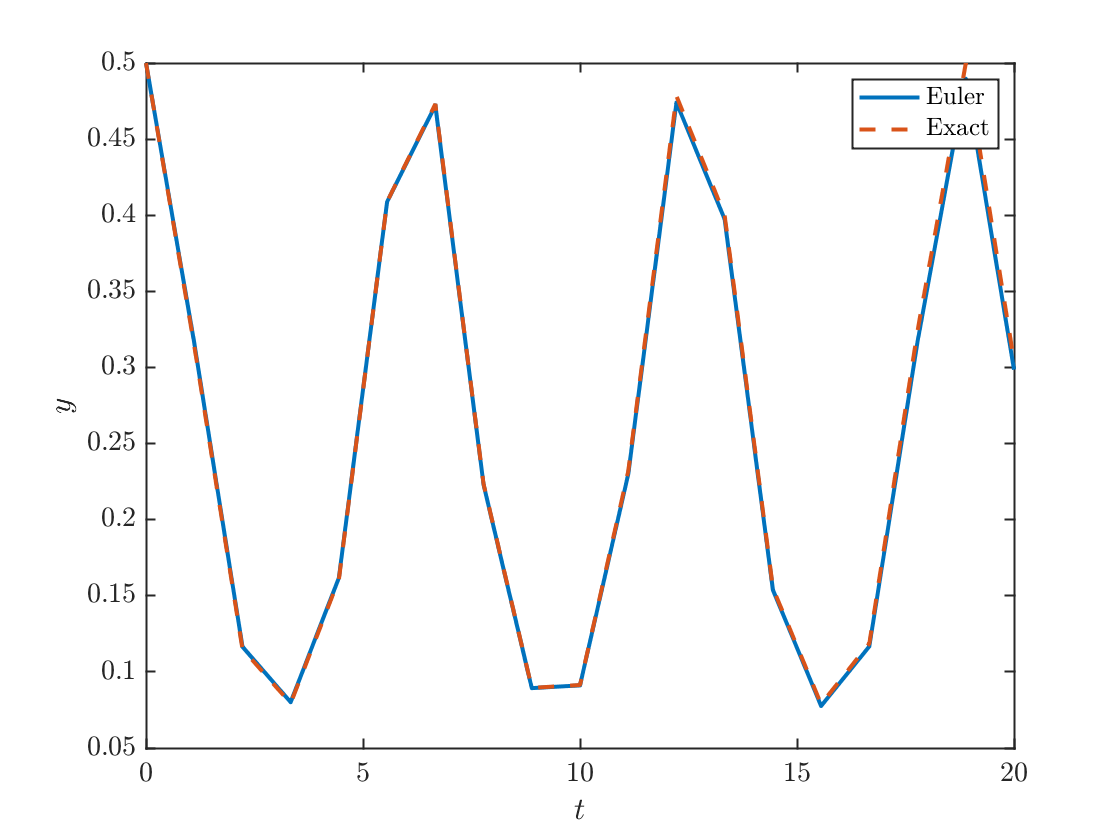error=abs(yX(end)-y(end));
fprintf('N = %i, error=%0.3f\n',N,error)
N = 18, error=0.006

## Show the Euler method is first order

N=1000;
h=(tEnd-tStart)/N;
t=linspace(tStart,tEnd,N+1);
y=zeros(1,N+1);
y(1)=y0;
for k=1:N
y(k+1)=eulerStep(@f,t(k),y(k),h);
end
error1 = abs(yExact(tEnd)-y(end));
fprintf('N = %i, error=%0.3f\n',N,error1)
N = 1000, error=0.019
N=2*N;
h=(tEnd-tStart)/N;
t=linspace(tStart,tEnd,N+1);
y=zeros(1,N+1);
y(1)=y0;
yX=yExact(t);
for k=1:N
y(k+1)=eulerStep(@f,t(k),y(k),h);
end
error2=abs(yExact(tEnd)-y(end));
fprintf('N = %i, error=%0.3f\n',N,error2)
N = 2000, error=0.010
errRatio=error1/error2;
fprintf('The error went down by a factor of %0.2f.\n',errRatio)
The error went down by a factor of 1.99.

## Show the Improved Euler method is second order

N=1000;
h=(tEnd-tStart)/N;
t=linspace(tStart,tEnd,N+1);
y=zeros(1,N+1);
y(1)=y0;
for k=1:N
y(k+1)=eulerImprovedStep(@f,t(k),y(k),h);
end
error1 = abs(yExact(tEnd)-y(end));
fprintf('N = %i, error=%0.d\n',N,error1)
N = 1000, error=9e-06
N=2*N;
h=(tEnd-tStart)/N;
t=linspace(tStart,tEnd,N+1);
y=zeros(1,N+1);
y(1)=y0;
yX=yExact(t);
for k=1:N
y(k+1)=eulerImprovedStep(@f,t(k),y(k),h);
end
error2=abs(yExact(tEnd)-y(end));
fprintf('N = %i, error=%0.3d\n',N,error2)
N = 2000, error=2.208e-06
errRatio=error1/error2;
fprintf('The error went down by a factor of %0.2f.\n',errRatio)
The error went down by a factor of 3.87.

## Show the Runge-Kutta method is fourth order

N=1000;
h=(tEnd-tStart)/N;
t=linspace(tStart,tEnd,N+1);
y=zeros(1,N+1);
y(1)=y0;
for k=1:N
y(k+1)=RKStep(@f,t(k),y(k),h);
end
error1 = abs(yExact(tEnd)-y(end));
fprintf('N = %i, error=%0.d\n',N,error1)
N = 1000, error=1e-10
N=2*N;
h=(tEnd-tStart)/N;
t=linspace(tStart,tEnd,N+1);
y=zeros(1,N+1);
y(1)=y0;
yX=yExact(t);
for k=1:N
y(k+1)=RKStep(@f,t(k),y(k),h);
end
error2=abs(yExact(tEnd)-y(end));
fprintf('N = %i, error=%0.3d\n',N,error2)
N = 2000, error=6.707e-12
errRatio=error1/error2;
fprintf('The error went down by a factor of %0.2f.\n',errRatio)
The error went down by a factor of 15.58.

## Functions used

function yprime= f(t,y)
yprime=(sin(t)*(y^3-y));
end
function y=yExact(t)
y=exp(cos(t))./sqrt(3*exp(2)+exp(2*cos(t)));
end
function yNew=eulerStep(f,t,y,h)
yNew=y+h*f(t,y);
end
function yNew=eulerImprovedStep(f,t,y,h)
k1=f(t,y);
k2=f(t+h,y+h*k1);
yNew=y+h*(k1+k2)/2;
end
function yNew=RKStep(f,t,y,h)
k1=f(t,y);
k2=f(t+h/2,y+h*k1/2);
k3=f(t+h/2,y+h*k2/2);
k4=f(t+h,y+h*k3);
yNew=y+h*(k1+2*k2+2*k3+k4)/6;
end